×

Search anything:

# Basic Bitwise Operations: AND, OR, XOR, NOT, LEFT and RIGHT SHIFT

#### bitwise operation and operation or operation not operation xor operation left shift operation right shift operationGet this book -> Problems on Array: For Interviews and Competitive Programming

It is a well-known fact that computers do not interpret numbers and other data like we do. They understand only binary, which is a dual system composed only of 0s and 1s. Programming languages provide an interface between the programmer and the system, converting whatever data we deal with into binary so that it can be processed by the computer, and then converting the result back into a form we can understand (usually decimal (base10) but also sometimes octal (base8) or hex (base16)).

In addition, most languages provide bitwise operators that allow you to perform certain operations at bit level. These operators and the supported operations are as follows:

### Bitwise AND (&)

This operator preforms a binary AND operation between the two operands. This means it compares the bits individually, the resultant bit mapping to 1 or true only if both bits fed to it are 1 as well.

Truth table:

A B A & B
0 0 0
0 1 0
1 0 0
1 1 1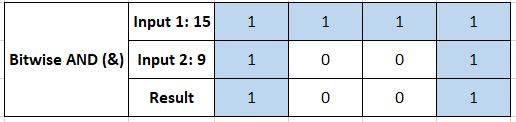### Bitwise OR (|)

This operator preforms a binary OR operation; the resultant bit mapping to 1 if one or more of the inputs to it is 1.

Truth table:

A B A OR B
0 0 0
0 1 1
1 0 1
1 1 1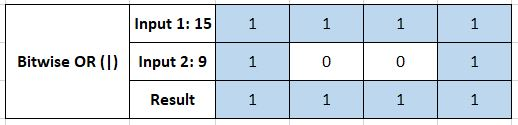### Bitwise XOR (^)

Performs the exclusive-OR or XOR operation; the resultant bit maps to 1 if both inputs have even number of ones (i.e. 0-1 or 1-0) but 0 otherwise.

Truth table:

A B A ^ B
0 0 0
0 1 1
1 0 1
1 1 0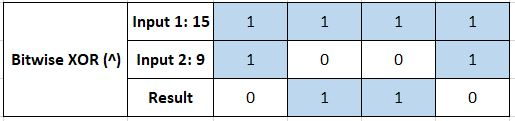### Bitwise Unary NOT (~)

Performs complementation or negation operation; inverts all the bits of the number, i.e. 0->1 and 1->0.

Truth table:

A ~ A
0 1
1 0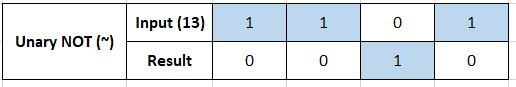### Left Shift (<<)

Performs a shift or rotation by a specified number of positions. Every bit is moved left n positions. Further, the MSB (Most Significant Bit) is shifted to the LSB (Least Significant Bit) for every rotation. It is the equivalent of multiplying the number by 2 n times.

Truth table:

A A << 1 A << 2
00010010 00100100 01001000
00111101 01111010 11110100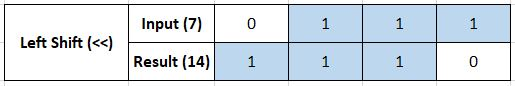### Right Shift (>>)

Every bit is moved right n positions. Further, in case of signed 2's complement numbers, the sign bit is moved into the MSB position. It is the equivalent of dividing the number by 2 n times.

A A >> 1 A >> 2
00010010 00001001 00000100
00111101 00011110 00001111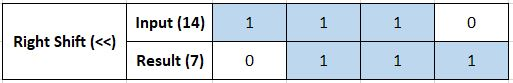#### OpenGenus Foundation

The official account of OpenGenus IQ backed by GitHub, DigitalOcean and Discourse

Improved & Reviewed by:

Basic Bitwise Operations: AND, OR, XOR, NOT, LEFT and RIGHT SHIFT